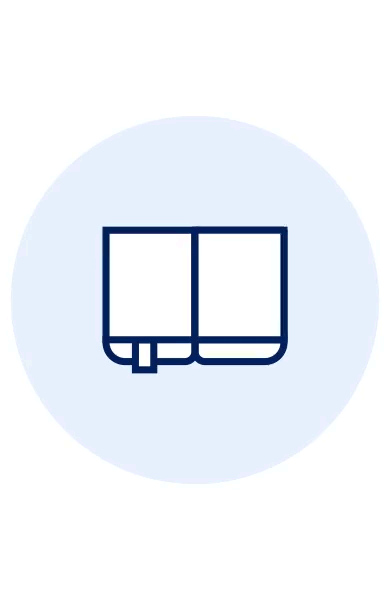MAI SUNT 00:00:00:00

MAI SUNT

X# Practical MATLAB Deep Learning

## De (autor): PaluszekHarness the power of MATLAB for deep-learning challenges. This book provides an introduction to deep learning and using MATLAB's deep-learning toolboxes. You'll see how these toolboxes provide the complete set of functions needed to implement all aspects of deep learning.
Along the way, you'll learn to model complex systems, including the stock market, natural language, and angles-only orbit determination. You'll cover dynamics and control, and integrate deep-learning algorithms and approaches using MATLAB. You'll also apply deep learning to aircraft navigation using images.
Finally, you'll carry out classification of ballet pirouettes using an inertial measurement unit to experiment with MATLAB's hardware capabilities.

What You Will Learn

Explore deep learning using MATLAB and compare it to algorithms
Write a deep learning function in MATLAB and train it with examples
Use MATLAB toolboxes related to deep learning
Implement tokamak disruption prediction

Who This Book Is For
Engineers, data scientists, and students wanting a book rich in examples on deep learning using MATLAB.
Citeste mai mult

transport gratuit

-20%

138.78Lei

173.48 Lei

Sau 13878 de puncteFiecare comanda noua reprezinta o investitie pentru viitoarele tale comenzi. Orice comanda plasata de pe un cont de utilizator primeste in schimb un numar de puncte de fidelitate, In conformitate cu regulile de conversiune stabilite. Punctele acumulate sunt incarcate automat in contul tau si pot fi folosite ulterior, pentru plata urmatoarelor comenzi.

Livrare in 3-5 saptamani

#### Descrierea produsului

Harness the power of MATLAB for deep-learning challenges. This book provides an introduction to deep learning and using MATLAB's deep-learning toolboxes. You'll see how these toolboxes provide the complete set of functions needed to implement all aspects of deep learning.
Along the way, you'll learn to model complex systems, including the stock market, natural language, and angles-only orbit determination. You'll cover dynamics and control, and integrate deep-learning algorithms and approaches using MATLAB. You'll also apply deep learning to aircraft navigation using images.
Finally, you'll carry out classification of ballet pirouettes using an inertial measurement unit to experiment with MATLAB's hardware capabilities.

What You Will Learn

Explore deep learning using MATLAB and compare it to algorithms
Write a deep learning function in MATLAB and train it with examples
Use MATLAB toolboxes related to deep learning
Implement tokamak disruption prediction

Who This Book Is For
Engineers, data scientists, and students wanting a book rich in examples on deep learning using MATLAB.
Citeste mai mult

Detaliile produsului

## De pe acelasi raft

Noi suntem despre carti, si la fel este si Newsletter-ul nostru.

Aboneaza-te si primesti un cupon de 10% pe care să-l folosesti la urmatoarea ta comanda! In plus, vei afla rapid care sunt promotiile zilei, noutatile si recomandarile noastre.